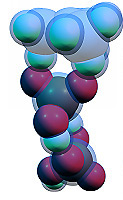## Choose orbitals window

In this form one chooses the orbitals, for which a cube (3-dimensional grid of orbital density values) should be built. If the checkbox "Save to cube file" is checked, these cubes are saved to a file after building. The textbox "Map points per angstrom" determines the density of the grid (total number of points is proportional to this value in third power). The textbox "Map size expansion" determines how long the cube would be extent over the molecule (i.e. the size of cube). The total number of points is shown at the left bottom corner; the time of building each orbital is proportional to this value. The button "Explicit coordinates" allows the exact number of grid points and its coordinates to be specified.

If one chooses the "Total SCF density" item, the program will calculate the total electron density (the sum of squares of all occupied alpha and beta MOs). If one chooses "Alpha - Beta SCF density", the program will calculate the spin density (sum of squares of all occupied alpha orbitals minus sum of squares of all occupied beta orbitals). If the item "Alha - Beta density of selected MOs" is chosen, the program will compute the sum of squares of all selected alpha orbitals minus sum of squares of all selected beta orbitals.

BACK